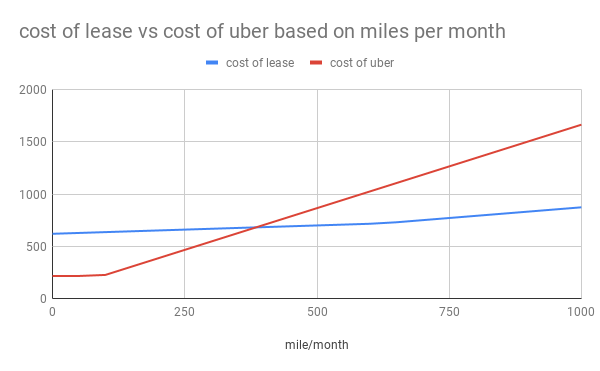# Cost of Leasing a Car vs Uber/Lyft

I have computed whether it is feasible to using Uber/Lyft instead of leasing/owning a car in the future.

# Assumption

Here are the assumptions/conditions for leasing a A Mercedes GLC 350e:

• costs you $450 per month for lease, which allows use to drive 7500 miles per month and charges you about 0.25 dollars per mile; • the car registration fee is california would be$604 per year;
• the car issurance is about $720 per half year • gas price is about$4 per gallon for premium and MPG is 25.

For using UberX, the price can be found from Uber’s website:

• booking fee is $2.2 per trip; • you need to pay$1.6 per mile;
• the minimum cost will be \$7.2 per trip.

Here we ignore the waiting time, which you need to pay Uber; and the parking fee, which need to pay if you drive by yourself.

# Result

The monthly cost of using UberX can be computed as:

=30*max(7.2, 2.2+1.6*A2/30)


The monthly cost of leasing a car can be computed as:

=604/12+720/6+450+min(7500/12,A2)*4/25+0.25*max(0,A2-7500/12)


The result is shown in the figure below: